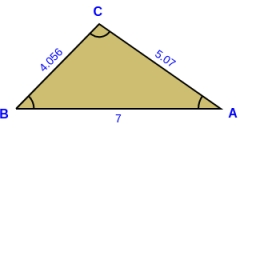# Magnitude 29891

The triangle ABC is the magnitude of the inner angle α 12 ° smaller than the angle β, and the angle γ is four times larger than the angle α. What size are these interior angles in the triangle?

A =  28
B =  40
C =  112

### Step-by-step explanation:

A=B-12
C = 4·A
A+B+C=180

A-B = -12
4A-C = 0
A+B+C = 180

Pivot: Row 1 ↔ Row 2
4A-C = 0
A-B = -12
A+B+C = 180

Row 2 - 1/4 · Row 1 → Row 2
4A-C = 0
-B+0.25C = -12
A+B+C = 180

Row 3 - 1/4 · Row 1 → Row 3
4A-C = 0
-B+0.25C = -12
B+1.25C = 180

Row 3 + Row 2 → Row 3
4A-C = 0
-B+0.25C = -12
1.5C = 168

C = 168/1.5 = 112
B = -12-0.25C/-1 = -12-0.25 · 112/-1 = 40
A = 0+C/4 = 0+112/4 = 28

A = 28
B = 40
C = 112

Our linear equations calculator calculates it.Did you find an error or inaccuracy? Feel free to write us. Thank you!

Tips for related online calculators
Do you have a system of equations and looking for calculator system of linear equations?